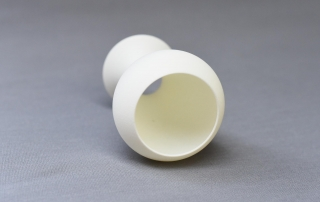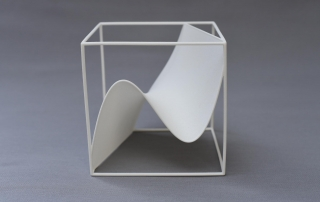•A math vase of degree 3 without bottom
Gallery

A math vase of degree 3 without bottom

A math vase of degree 3 without bottom. It has been created by rotating a graph of a polynomial of degree 3 about an axis.

•A math vase of degree 3
Gallery

A math vase of degree 3

A math vase of degree 3. It has been creating by rotating a graph of a polynomial of degree 3 about an axis.

•A 3d graph of a cubic function
Gallery

A 3d graph of a cubic function

This visualizes a 1-parameter family of cubic functions or a 3d graph of a function in one variable in a 3d-coordinate system.